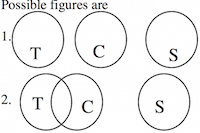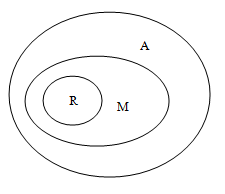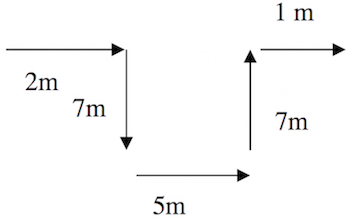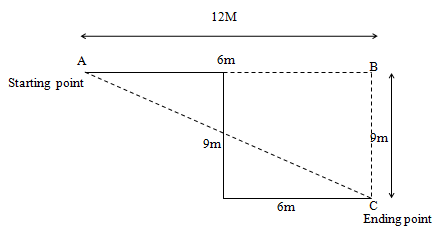# Zensar Reasoning Question

DIRECTION for questions 1 to 2:In each question below are two/three statements followed by two conclusions numbered I and II. You have to take the two/three given statements to be true even if they seem to be at variance from commonly known facts and then decide which of the given conclusions logically follows from the given statements disregarding commonly known facts.
1. Statements: No toffee is coffee. No sweet is toffee.
Conclusions: I. No coffee is sweet.
II. All sweets are coffee.
1. only conclusion I follows.
2. only conclusion II follows.
3. either conclusion I or conclusion II follows.
4. neither conclusion I nor conclusion II follows.
5. both conclusion I and conclusion II follows.Conclusions I. No coffee is sweet. This statement is not true as is seen from the 2nd figure shown above.
Conclusions II. All sweets are coffee. This is definitely false.
So, neither conclusion I nor conclusion II follows.
2. Statements: All medals are awards. All rewards are medals.
Conclusions: I. All rewards are awards
II. All awards are medals
1. only conclusion I follows.
2. only conclusion II follows.
3. either conclusion I or conclusion II follows.
4. neither conclusion I nor conclusion II follows.
5. both conclusion I and conclusion II follows.So all rewards are awards
So, conclusion I is true.
II. All awards are medals.
This is clearly false.
So, only conclusion I follows.
1. In a certain code PAUSE is written as OBVTD and SHIFT is written as RIJGS. How will THINK be written in the same code?
1. UGHML
2. SIJOJ
3. SILOJ
4. SIJQJ
5. SIJOL
First and last letters are changing into previous one and other letters are changing into next letters.
So, THINK will change into SIJOJ.
2. Ajay walked 2m towards east, took a right turn and walked 7m. He then took a left turn and walked 5m before taking a left turn and walking 7m. He then took a final right turn and walked 1m before stopping. How far is Ajay from the starting point ?
1. 8m
2. 7m
3. 6m
4. 5m
5. 9mSo distance from starting point is 8m.
DIRECTION for questions 5 to 7: Study the following information to answer the given questions:
Eight people are sitting in two parallel rows containing four people each, in such a way that there is an equal distance between adjacent persons. In row 1- A, B, C and D are seated (but not necessarily in the same order) and all of them are facing south. In row 2 - E, F, G and H are seated (but not necessarily in the same order) and all of them are facing north. Therefore, in the given seating arrangement each member seated in a row faces another member of the other row.G sits second to right of E. The one who faces E sits to the immediate right of C. A faces the immediate neighbour of E. H sits to immediate left of the person who faces D. H does not sit at an extreme end of the line.
3. Who amongst the following faces G ?
1. A
2. B
3. C
4. D
5. Cannot be determined
We have,
G sits second to right of E i.e. _ E _ G
The one who faces E sits to the immediate right of C i.e.
C _ _ _
_ E _ G
A faces the immediate neighbour of E i.e.
C _ A _
_ E _ G
H does not sit at an extreme end of the line i.e. C _ A _
_ E H G
H sits to immediate left of the person who faces D i.e.
C _ A D
F E H G
Pattern is
1st row C B A D
2nd row F E H G
So, G faces D.
4. Which of the following is true regarding B?
1. B sits exactly between C and D
2. D sits second to right of B
3. B sits at an extreme end of the line
4. B is an immediate neighbour of the person who faces F
5. None is true
We have, G sits second to right of E i.e. _ E _ G
The one who faces E sits to the immediate right of C i.e.
C _ _ _
_ E _ G
A faces the immediate neighbour of E i.e.
C _ A _
_ E _ G
H does not sit at an extreme end of the line i.e.
C _ A _
_ E H G
H sits to immediate left of the person who faces D i.e.
C _ A D
F E H G
Pattern is
1st row C B A D
2nd row F E H G
Only 4th option satisfies condition.
5. Four of the following five are alike in a certain way based on the given seating arrangement and thus form a group. Which is the one that does not belong to that group ?
1. E
2. D
3. F
4. G
5. C
G sits second to right of E i.e. _ E _ G
The one who faces E sits to the immediate right of C i.e.
C _ _ _
_ E _ G
A faces the immediate neighbour of E i.e.
C _ A _
_ E _ G
H does not sit at an extreme end of the line i.e.
C _ A _
_ E H G
H sits to immediate left of the person who faces D i.e.
C _ A D
F E H G
Pattern is
1st row C B A D
2nd row F E H G
All of them are at extreme positions except E.
So, correct answer is option A.
DIRECTION for question 8: Solve the following question and mark the best possible option.
6. Statements: B < A < C; A> D ≤ E
Conclusions: I. B ≤ E II. C > E
1. only conclusion I follows.
2. only conclusion II follows.
3. either conclusion I or conclusion II follows.
4. neither conclusion I nor conclusion II follows.
5. both conclusion I and conclusion II follows.
From the given statements, it can be concluded that B < A > D ≤ E.
Thus, according to above, either B ≤ E or B > E.
Similarly, according to given statements E ≥ D
< A < C, either C = E or C < E or C > E.
As none of them is definite the answer will be fourth option.
7. How many such digits are there in the number 3246759, each of which will occupy the same position when rearranged in ascending order as in the number?
1. None
2. One
3. Two
4. Three
5. More than three